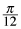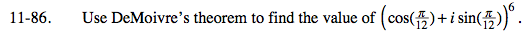Home > PC > Chapter 11 > Lesson 11.2.2 > Problem11-86

11-86.

Use DeMoivre’s theorem to find the value of (cos() + i sin())6. Homework Help ✎Using the theorem, r = 1, n = 6, and θ = π/12.

$z^6=1^6\left( \cos\frac{6\pi}{12}+i\sin\frac{6\pi}{12} \right)$